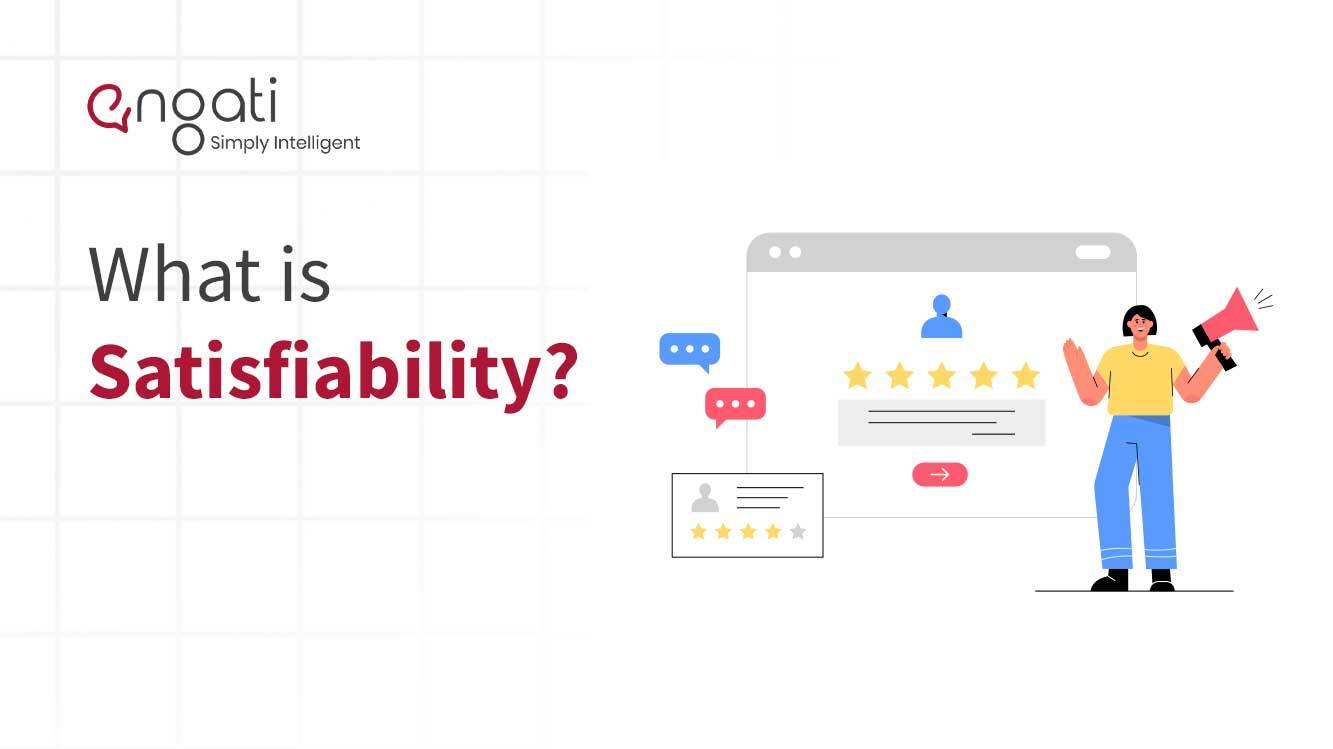# Satisfiability## What is satisfiability?

In mathematical logic, particularly, first-order logic and propositional calculus, satisfiability and validity are elementary concepts of semantics. A formula is satisfiable if there exists a model that makes the formula true. A formula is valid if all models make the formula true. The opposites of these concepts are unsatisfiability and invalidity, that is, a formula is unsatisfiable if none of the models make the formula true, and invalid if some such models make the formula false. These four concepts are related to each other in a manner exactly analogous to Aristotle's square of opposition.

The four concepts can be raised to apply to whole theories:

• A theory is satisfiable (valid) if one (all) of the interpretations make(s) each of the axioms of the theory true
• A theory is unsatisfiable (invalid) if all (one) of the interpretations make(s) one of the axioms of the theory false.

It is also possible to consider only interpretations that make all of the axioms of a second theory true. This generalization is commonly called satisfiability modulo theories.

The question whether a sentence in propositional logic is satisfiable is a decidable problem, or a boolean satisfiability problem. In general, the question whether a sentence of first-order logic is satisfiable is not decidable. In universal algebra and equational theory, the methods of term rewriting, congruence closure and unification are used to attempt to decide satisfiability. Whether a particular theory is decidable or not depends whether the theory is variable-free or on other conditions

## Which areas are satisfiability applied in?

In practice, many automated reasoning problems in Propositional Logic are first reduced to satisfiability problems and then by using a satisfiability solver. Today, SAT solvers are commonly used in hardware design, software analysis, planning, mathematics, security analysis, and many other areas.Request a Demo!

Get started on Engati with the help of a personalised demo.

Thanks for the information.
We will be shortly getting in touch with you.
For any other query reach out to us on contact@engati.comI am looking for a conversational AI engagement solution for the web and other channels.I would like for a conversational AI engagement solution for WhatsApp as the primary channelI am an e-commerce store with Shopify. I am looking for a conversational AI engagement solution for my businessI am looking to partner with Engati to build conversational AI solutions for other businessesYou're a step away from building your Al chatbot

How many customers do you expect to engage in a month?

Less Than 2000

2000-5000

More than 5000Thanks for the information.

We will be shortly getting in touch with you.Thanks for the information.
We will be shortly getting in touch with you.
Oops! Looks like there is a problem.
Never mind, drop us a mail at contact@engati.com

<script type="application/ld+json">
{
"@context": "https://schema.org",
"@type": "FAQPage",
"mainEntity": [{
"@type": "Question",
"name": "What is satisfiability?",